# P-value functions: Tutorial using the `pvaluefunctions` package

## Accompanying paper

We published an accompanying paper to illustrate the use of p-value functions:

Infanger D, Schmidt-Trucksäss A. (2019): P value functions: An underused method to present research results and to promote quantitative reasoning. Statistics in Medicine, 38: 4189-4197. doi: 10.1002/sim.8293.

### Recreation of the graphics in the paper

The code and instructions to reproduce all graphics in our paper can be found in the following GitHub repository: https://github.com/DInfanger/pvalue_functions

## Overview

This vignette shows how to use the `pvaluefunctions` package wich contains an R function to create graphics of p-value functions, confidence distributions, confidence densities, or the Surprisal value (S-value) (Greenland 2019).

## Installation

Install (`install.packages("pvaluefunctions")`) and load the package from CRAN.

``library(pvaluefunctions)``

## Dependencies

The function depends on the following R packages:

• ggplot2 (at least version 3.2.1)
• scales
• zipfR

## Usage

There is only one function needed to create the plots: `conf_dist()`. The function has the following arguments:

• `estimate`: Numerical vector containing the estimate(s).
• `n`: Numerical vector containing the sample size(s). Required for correlations, variances, proportions and differences between proportions. Must be equal the number of estimates.
• `df`: Numerical vector containing the degrees of freedom. Required for statistics based on the t-distribution (e.g. linear regression) and t-tests. Must be equal the number of estimates.
• `stderr`: Numerical vector containing the standard error(s) of the estimate(s). Required for statistics based on the t-distribution (e.g. linear regression) and the normal distribution (e.g. logistic regression). Must be equal the number of estimate(s).
• `tstat`: Numerical vector containing the t-statistic(s). Required for t-tests (means and mean differences). Must be equal the number of estimates.
• `type`: String indicating the type of the estimate. Must be one of the following: `ttest`, `linreg`, `gammareg`, `general_t`, `logreg`, `poisreg`, `coxreg`, `general_z`, `pearson`, `spearman`, `kendall`, `var`, `prop`, `propdiff`.
• `plot_type`: String indicating the type of plot. Must be one of the following: `cdf` (confidence distribution), `pdf` (confidence density), `p_val` (p-value function, the default), `s_val` (Surprisal). For differences between independent proportions, only p-value functions and Surprisal value functions are available.
• `n_values` (optional): Integer indicating the number of points that are used to generate the graphics. The higher this number, the higher the computation time and resolution.
• `est_names` (optional): String vector indicating the names of the estimate(s). Must be equal the number of estimates.
• `conf_level` (optional): Numerical vector indicating the confidence level(s). Bust be between 0 and 1.
• `null_values` (optional): Numerical vector indicating the null value(s) in the plot on the untransformed scale. For example: If you want to plot odds ratios from logistic regressions, the `null_values` have to be given on the log-odds scale. If x limits are specified with `xlim`, all null values outside of the specified x limits are ignored for plotting and a message is printed.
• `trans` (optional): String indicating the transformation function that will be applied to the estimates and confidence curves. For example: `"exp"` for an exponential transformation of the log-odds in logistic regression.
• `alternative`: String indicating if the confidence level(s) are two-sided or one-sided. Must be one of the following: `two_sided`, `one_sided`.
• `log_yaxis`: Logical. Indicating if a portion of the y-axis should be displayed on the logarithmic scale.
• `cut_logyaxis`: Numerical value indicating the threshold below which the y-axis will be displayed logarithmically. Must lie between 0 and 1.
• `xlim` (optional) Optional numerical vector of length 2 (x1, x2) indicating the limits of the x-axis on the untransformed scale. For example: If you want to plot p-value functions for odds ratios from logistic regressions, the limits have to be given on the log-odds scale. Note that x1 > x2 is allowed but then x2 will be the left limit and x1 the right limit (i.e. the limits are sorted before plotting). Null values (specified in `null_values`) that are outside of the specified limits are ignored and a message is printed.
• `together`: Logical. Indicating if graphics for multiple estimates should be displayed together or on separate plots.
• `nrow`: (optional) Integer greater than 0 indicating the number of rows when `together = FALSE` is specified for multiple estimates. Used in `facet_wrap` in ggplot2.
• `ncol`: (optional) Integer greater than 0 indicating the number of columns when `together = FALSE` is specified for multiple estimates. Used in `facet_wrap` in ggplot2.
• `plot_p_limit`: Numerical value indicating the lower limit of the y-axis. Must be greater than 0 for a logarithmic scale (i.e. `log_yaxis = TRUE`). The default is to omit plotting p-values smaller than 1 - 0.999 = 0.001.
• `plot_counternull`: Logical. Indicating if the counternull should be plotted as a point. Only available for -value functions and s-value functions. Counternull values that are outside of the plotted functions are not shown.
• `title` (optional): String containing a title for the plot.
• `xlab` (optional): String indicating the label of the x-axis.
• `ylab` (optional): String containing a title for the primary (left) y-axis.
• `ylab_sec` (optional): String containing a title for the secondary (right) y-axis.
• `inverted` Logical. Indicating the orientation of the P-value function (`p_val`), S-value function (`s_val`) and confidence distribution (`cdf`). By default (i.e. `inverted = FALSE`) small P-values are plotted at the bottom and large ones at the top. By setting `inverted = TRUE`, the y-axis is invertedd. Ignored for confidence densities.
• `x_scale` String indicating the scaling of the x-axis. The default is to scale the x-axis logarithmically if the transformation specified in `trans` is “exp” (exponential) and linearly otherwise. The option `linear` (can be abbreviated) forces a linear scaling and the option `logarithm` (can be abbreviated) forces a logarithmic scaling.
• `plot` Logical. Should a plot be created (`TRUE`, the default) or not (`FALSE`). `FALSE` can be useful if users want to create their own plots. If `FALSE`, no ggplot2 object is returned.

### Required arguments for different estimate types

• t-tests: `estimate`, `df`, `tstat`.
• Linear regression, Gamma regression, general estimates with inference based on the t-distribution: `estimate`, `df`, `stderr`.
• Logistic regression, Poisson regression, Cox regression, general estimates with inference based on the normal distribution: `estimate`, `stderr`.
• Correlation coefficients (Pearson, Spearman, Kendall), proportions, difference between proportions, variances: `estimate`, `n`.

### Returned values

The main function `conf_dist()` returns five objects in a list:

• `res_frame`: A data frame containing the values used to construct the plot. It contains the following variables: `values` contain the values of the effect (e.g. mean difference, odds ratio etc.), `conf_dist` the values for the confidence distribution, `conf_dens` the values for the confidence density, `p_two` the values for the two-sided p-value function, `p_one` the values for the one-sided p-value function, `s_val` the S-value (surprisal) of the two-sided p-values, `variable` the name of the estimate, `hypothesis` indicating the alternative hypothesis if one-sided p-value functions were specified and `counternull` containing the counternull values.
• `conf_frame`: A data frame containing the confidence intervals for the specified confidence levels for all estimates.
• `counternull_frame`: A data frame containing the counternull values for the specified null values (see Rosenthal & Rubin (1994) for more information about the counternull).
• `point_est`: A data frame containing the point estimates for all estimates. The point estimates correspond to the mean, median or mode of the confidence density (see Xie & Singh (2013) for more information). Estimates are produced using numerical procedures: Increase the number of points `n_values` for higher numerical precision.
• `plot`: A ggplot2 plot object (only returned if the option `plot = TRUE` was specified).

## Examples

### Two-sample t-test with unequal variances (Welch-Test)

``````#-----------------------------------------------------------------------------
# T-Test
#-----------------------------------------------------------------------------

with(sleep, mean(extra[group == 1])) - with(sleep, mean(extra[group == 2]))
#>  -1.58
t.test(extra ~ group, data = sleep, var.equal = FALSE)
#>
#>  Welch Two Sample t-test
#>
#> data:  extra by group
#> t = -1.8608, df = 17.776, p-value = 0.07939
#> alternative hypothesis: true difference in means is not equal to 0
#> 95 percent confidence interval:
#>  -3.3654832  0.2054832
#> sample estimates:
#> mean in group 1 mean in group 2
#>            0.75            2.33

#-----------------------------------------------------------------------------
# Create p-value function
#-----------------------------------------------------------------------------

res <- conf_dist(
estimate = c(-1.58)
, df = c(17.77647)
, tstat = c(-1.860813)
, type = "ttest"
, plot_type = "p_val"
, n_values = 1e4L
# , est_names = c("")
, conf_level = c(0.95, 0.90, 0.80)
, null_values = c(0)
, trans = "identity"
, alternative = "two_sided"
, log_yaxis = FALSE
, cut_logyaxis = 0.05
, xlab = "Mean difference (group 1 - group 2)"
, together = FALSE
, plot_p_limit = 1 - 0.999
, plot_counternull = TRUE
, x_scale = "line"
, plot = TRUE
)``````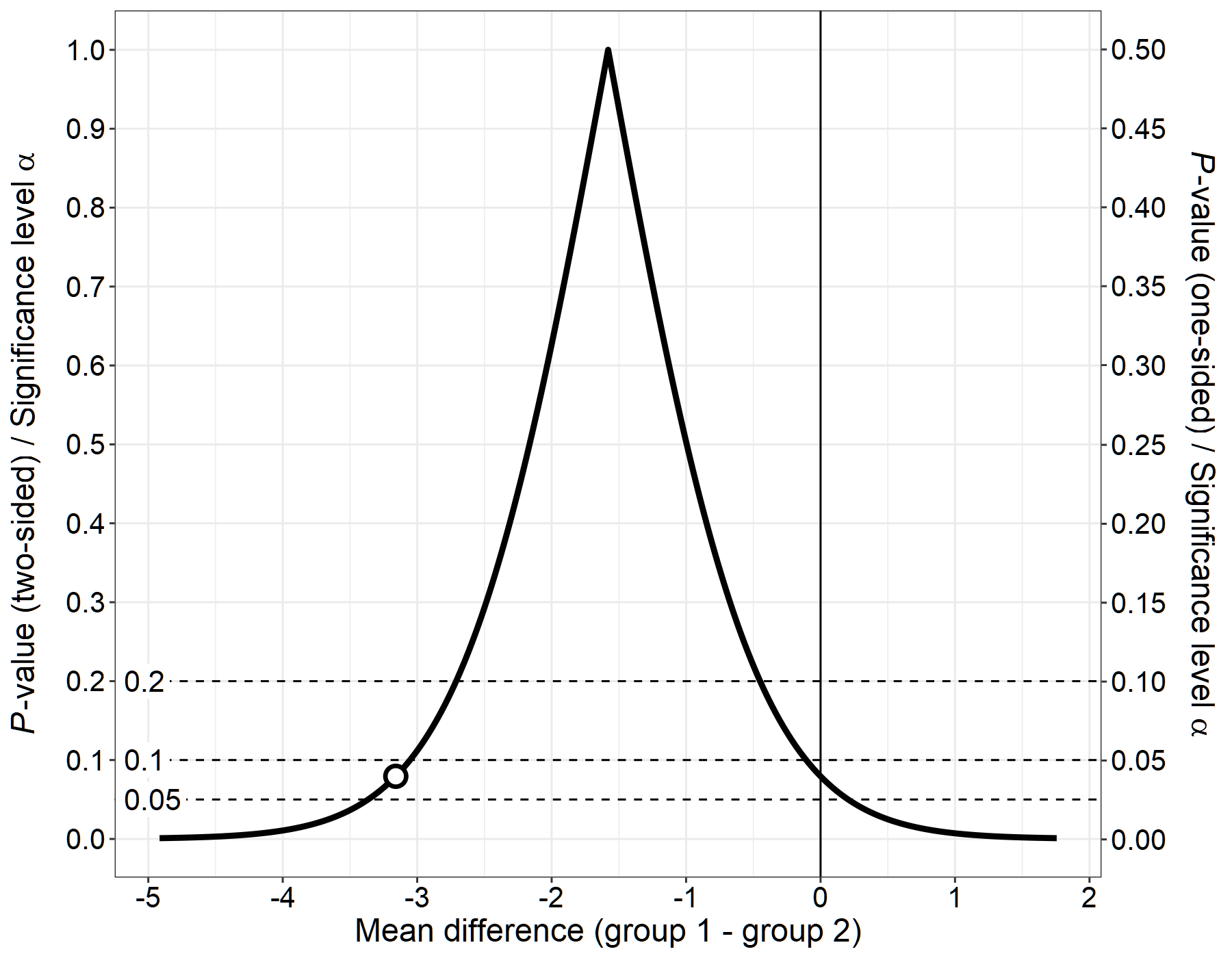### Single coefficient from a linear regression model

#### P-value function

Because it’s difficult to see very small p-values in the graph, you can set the option `log_yaxis = TRUE` so that p-values (i.e. the y-axes) below the value set in `cut_logyaxis` will be plotted on a logarithmic scale. This will make it much easier to see small p-values but has the disadvantage of creating a “kink” in the p-value function which is a pure artifact and puts an undue emphasis on the specified cutoff.

``````#-----------------------------------------------------------------------------
# Model
#-----------------------------------------------------------------------------

mod <- lm(Infant.Mortality~Agriculture + Fertility + Examination, data = swiss)

summary(mod)
#>
#> Call:
#> lm(formula = Infant.Mortality ~ Agriculture + Fertility + Examination,
#>     data = swiss)
#>
#> Residuals:
#>     Min      1Q  Median      3Q     Max
#> -8.5375 -1.4021 -0.0066  1.7381  5.9150
#>
#> Coefficients:
#>             Estimate Std. Error t value Pr(>|t|)
#> (Intercept) 11.01896    4.47291   2.463  0.01784 *
#> Agriculture -0.02143    0.02394  -0.895  0.37569
#> Fertility    0.13115    0.04145   3.164  0.00285 **
#> Examination  0.04913    0.08351   0.588  0.55942
#> ---
#> Signif. codes:  0 '***' 0.001 '**' 0.01 '*' 0.05 '.' 0.1 ' ' 1
#>
#> Residual standard error: 2.645 on 43 degrees of freedom
#> Multiple R-squared:  0.2291, Adjusted R-squared:  0.1753
#> F-statistic:  4.26 on 3 and 43 DF,  p-value: 0.01014

#-----------------------------------------------------------------------------
# Create p-value function
#-----------------------------------------------------------------------------

res <- conf_dist(
estimate = c(-0.02143)
, df = c(43)
, stderr = (0.02394)
, type = "linreg"
, plot_type = "p_val"
, n_values = 1e4L
# , est_names = c("")
, conf_level = c(0.95, 0.90, 0.80)
, null_values = c(0)
, trans = "identity"
, alternative = "two_sided"
, log_yaxis = TRUE
, cut_logyaxis = 0.05
, xlab = "Coefficient Agriculture"
, xlim = c(-0.12, 0.065)
, together = FALSE
, plot_p_limit = 1 - 0.999
, plot_counternull = FALSE
, plot = TRUE
)``````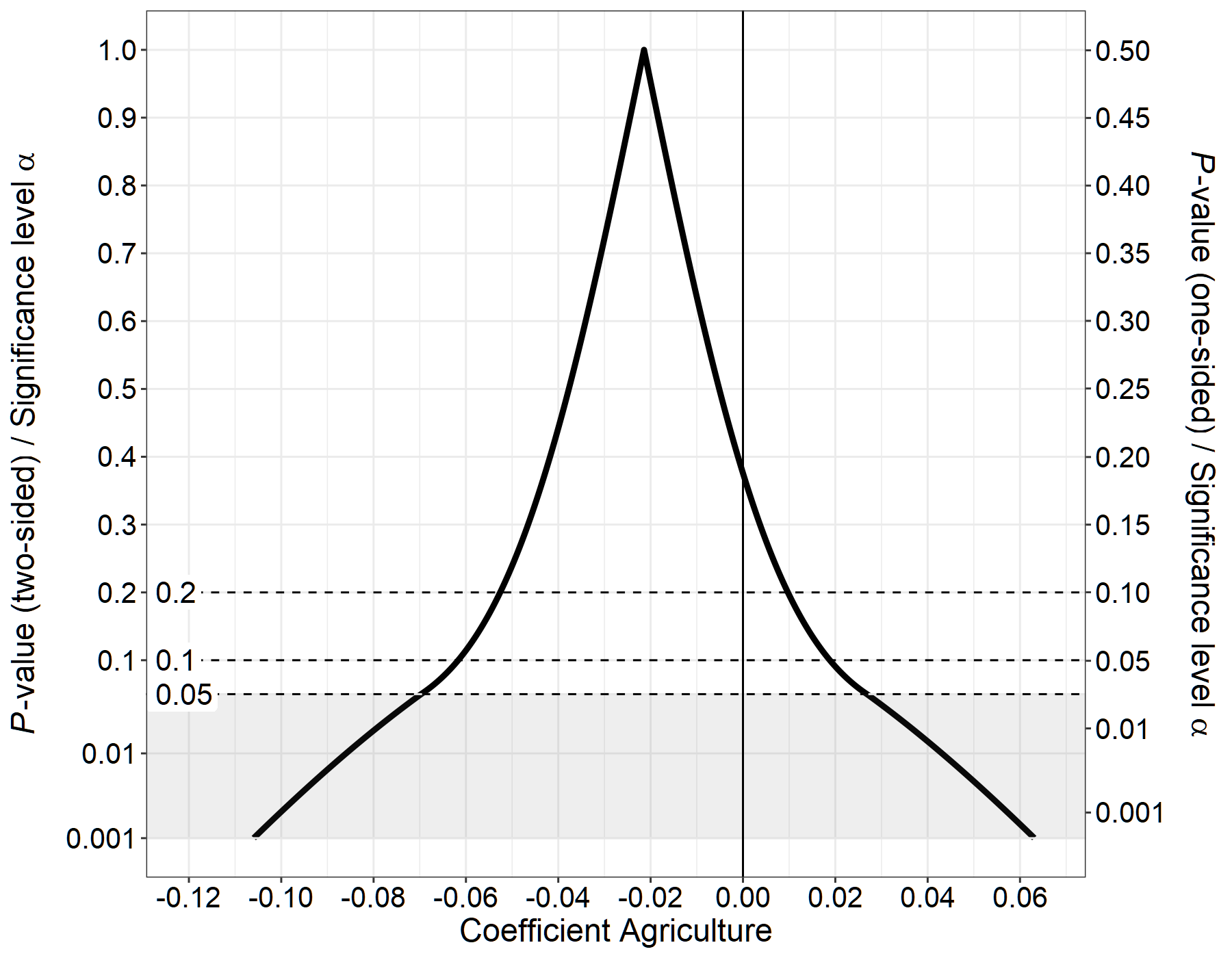#### Confidence distribution

``````res <- conf_dist(
estimate = c(-0.02143)
, df = c(43)
, stderr = (0.02394)
, type = "linreg"
, plot_type = "cdf"
, n_values = 1e4L
# , est_names = c("")
, conf_level = c(0.95, 0.90, 0.80)
, null_values = c(0)
, trans = "identity"
, alternative = "two_sided"
# , log_yaxis = TRUE
# , cut_logyaxis = 0.05
, xlab = "Coefficient Agriculture"
, xlim = c(-0.12, 0.065)
, together = FALSE
# , plot_p_limit = 1 - 0.999
, plot_counternull = FALSE
)``````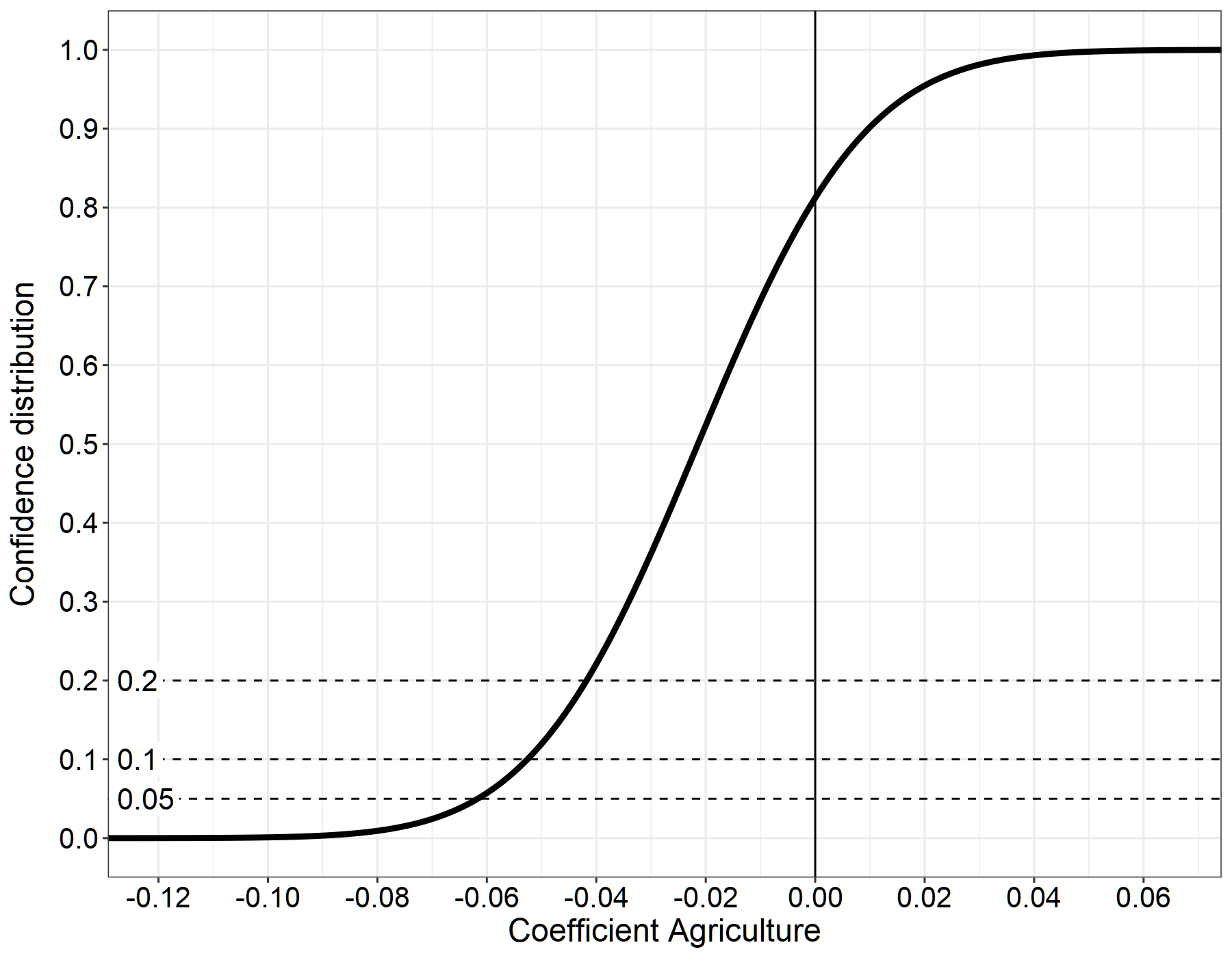The point where the confidence distribution is \(0.5\) is the median unbiased estimator (see Xie & Singh (2013) for a review and proofs).

### Multiple coefficients from a linear regression model

#### P-value functions

``````res <- conf_dist(
estimate = c(0.13115, 0.04913)
, df = c(43, 43)
, stderr = c(0.04145, 0.08351)
, type = "linreg"
, plot_type = "p_val"
, n_values = 1e4L
, est_names = c("Fertility", "Examination")
, conf_level = c(0.95, 0.90, 0.80)
, null_values = c(0)
, trans = "identity"
, alternative = "two_sided"
, log_yaxis = FALSE
, cut_logyaxis = 0.05
, xlab = "Regression coefficients"
, together = TRUE
, plot_p_limit = 1 - 0.999
, plot_counternull = FALSE
, inverted = FALSE
)``````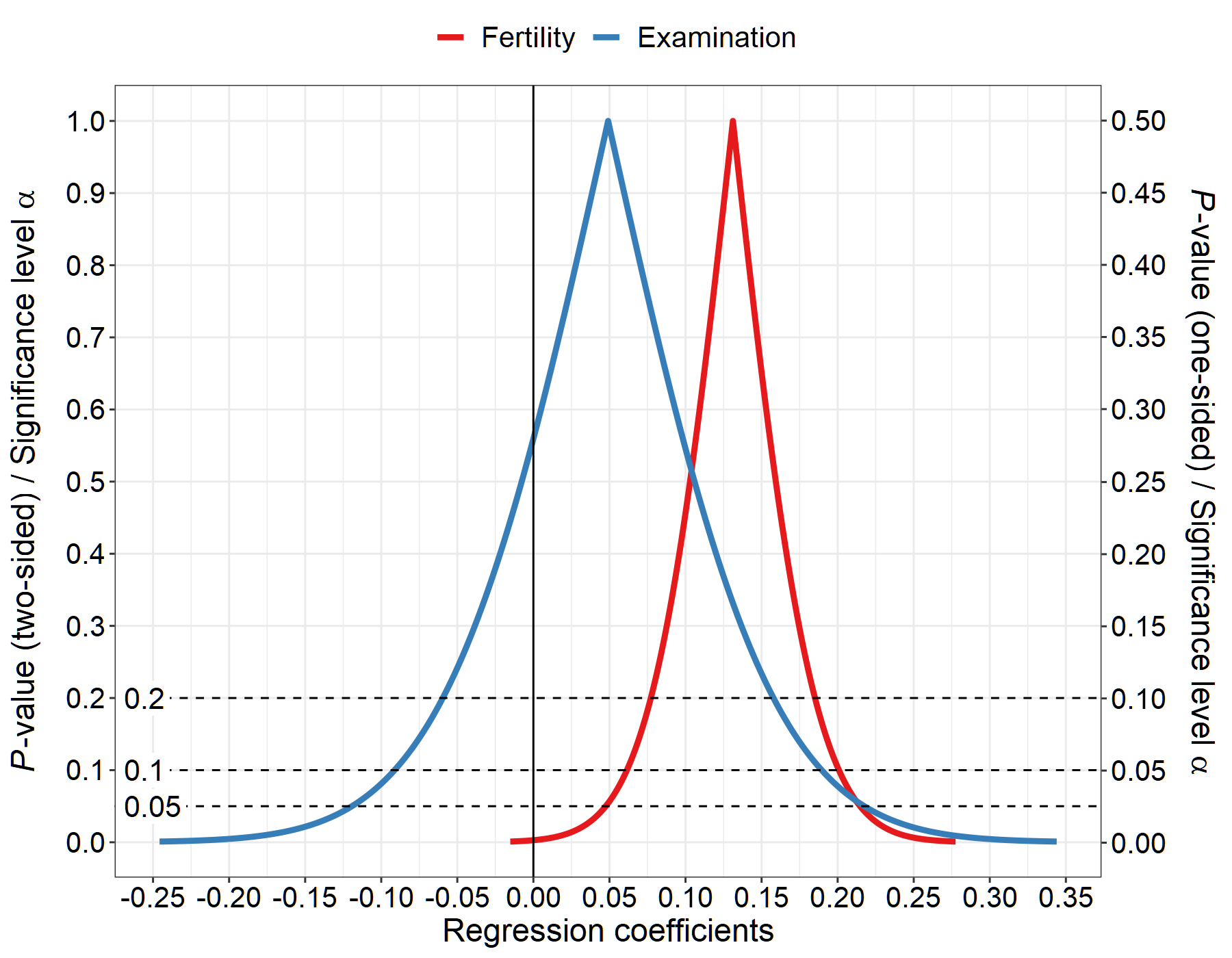#### Surprisal values

``````res <- conf_dist(
estimate = c(0.13115, 0.04913)
, df = c(43, 43)
, stderr = c(0.04145, 0.08351)
, type = "linreg"
, plot_type = "s_val"
, n_values = 1e4L
, est_names = c("Fertility", "Examination")
, conf_level = c(0.95, 0.90, 0.80)
, null_values = c(0)
, trans = "identity"
, alternative = "two_sided"
# , log_yaxis = TRUE
# , cut_logyaxis = 0.05
, xlab = "Regression coefficients"
, together = TRUE
, plot_p_limit = 1 - 0.999
, plot_counternull = TRUE
)``````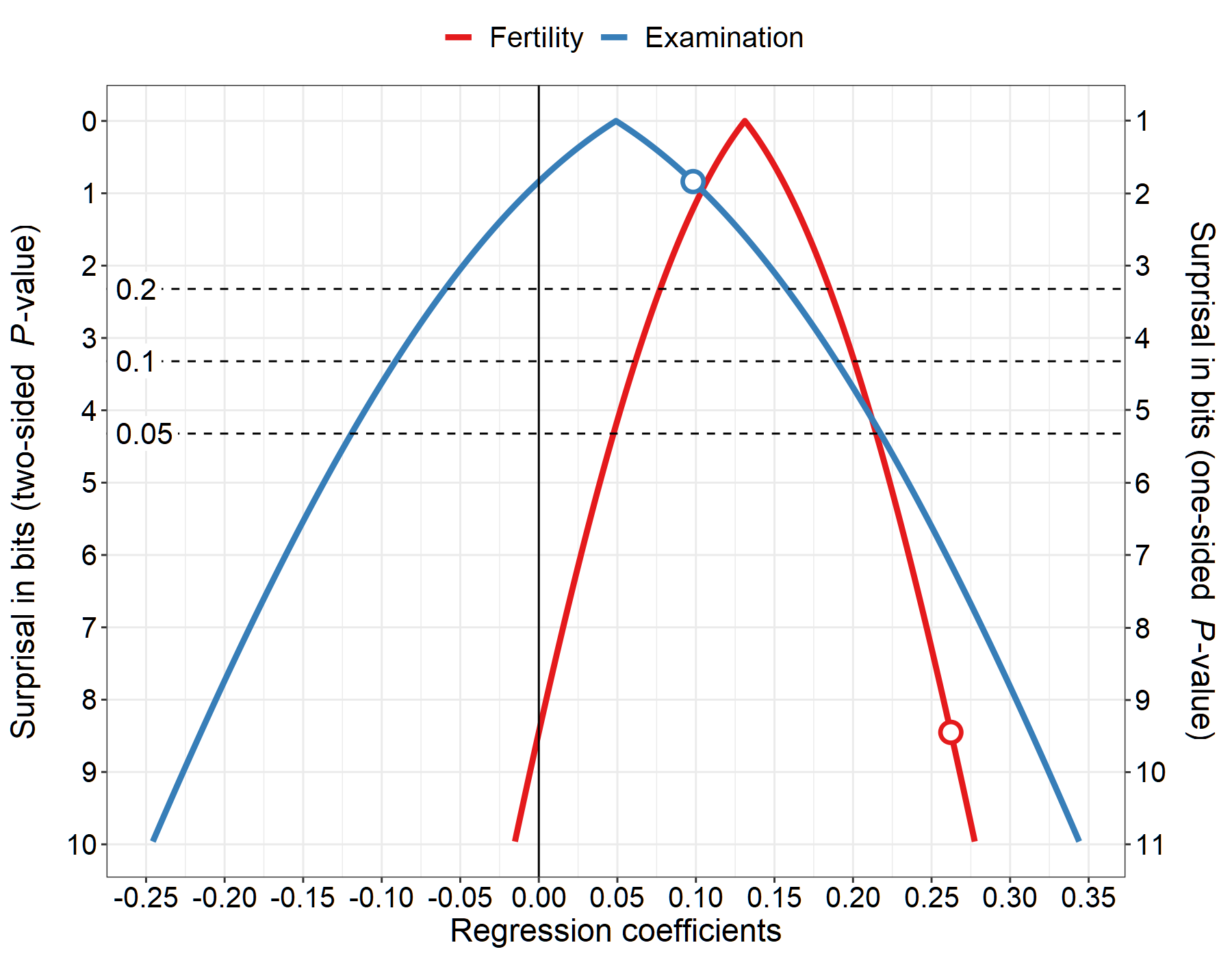### Pearson correlation coefficient (one-sided)

``````#-----------------------------------------------------------------------------
# Calculate Pearson's correlation coefficient
#-----------------------------------------------------------------------------

cor.test(swiss\$Fertility, swiss\$Agriculture, alternative = "two.sided", method = "pearson")
#>
#>  Pearson's product-moment correlation
#>
#> data:  swiss\$Fertility and swiss\$Agriculture
#> t = 2.5316, df = 45, p-value = 0.01492
#> alternative hypothesis: true correlation is not equal to 0
#> 95 percent confidence interval:
#>  0.07334947 0.58130587
#> sample estimates:
#>       cor
#> 0.3530792

#-----------------------------------------------------------------------------
# Create p-value function
#-----------------------------------------------------------------------------

res <- conf_dist(
estimate = c(0.3530792)
, n = 47
, type = "pearson"
, plot_type = "p_val"
, n_values = 1e4L
# , est_names = c("")
, conf_level = c(0.95, 0.90, 0.80)
, null_values = c(0)
, trans = "identity"
, alternative = "one_sided"
, log_yaxis = FALSE
, cut_logyaxis = 0.05
, xlab = "Pearson correlation"
, together = TRUE
, plot_p_limit = 1 - 0.999
, plot_counternull = FALSE
)``````